Next: Planetary orbits Up: Keplerian orbits Previous: Elliptic orbits

# Orbital elements

The previous analysis suffices when considering a single planet orbiting around the Sun. However, it becomes inadequate when dealing with multiple planets whose orbital planes and perihelion directions do not necessarily coincide. Incidentally, for the time being, we are neglecting interplanetary gravitational interactions, which allows us to assume that each planet executes an independent Keplerian elliptical orbit about the Sun.

Let us characterize all planetary orbits using a common Cartesian coordinate system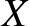,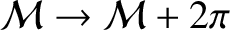,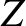, centered on the Sun. (See Figure 4.6.) The-plane defines a reference plane, which is chosen to be the ecliptic plane (i.e., the plane of the Earth's orbit), with the-axis pointing towards the ecliptic north pole (i.e., the direction normal to the ecliptic plane in a northward sense). Likewise, the-axis defines a reference direction, which is chosen to point in the direction of the vernal equinox (i.e., the point in the Earth's sky at which the apparent orbit of the Sun passes through the extension of the Earth's equatorial plane from south to north). Suppose that the plane of a given planetary orbit is inclined at an angleto the reference plane. The point at which this orbit crosses the reference plane in the direction of increasingis termed its ascending node. The anglesubtended between the reference direction and the direction of the ascending node is termed the longitude of the ascending node. Finally, the angle,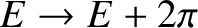, subtended between the direction of the ascending node and the direction of the orbit's perihelion is termed the argument of the perihelion.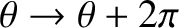Let us define a second Cartesian coordinate system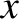,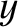,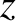, also centered on the Sun. Let the-plane coincide with the plane of a particular planetary orbit, and let the-axis point towards the orbit's perihelion point. Clearly, we can transform from the,,system to the,,system via a series of three rotations of the coordinate system: first, a rotation through an angleabout the-axis (looking down the axis); second, a rotation through an angleabout the new-axis; and finally, a rotation through an angleabout the new-axis. It thus follows from standard coordinate transformation theory (see Section A.6) that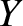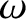(4.71)

However,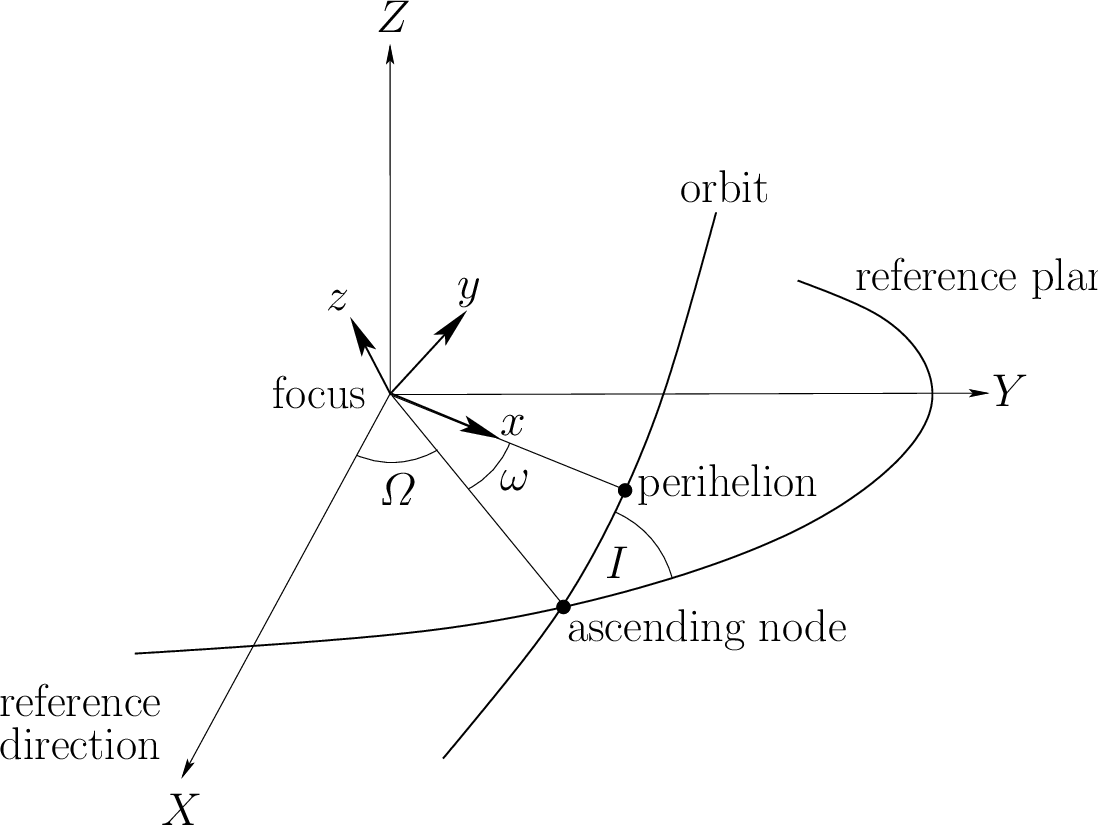,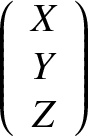, and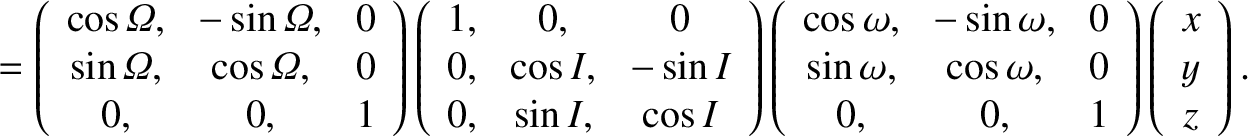. Hence,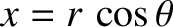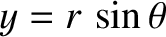(4.72)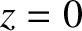(4.73) and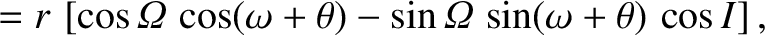(4.74)

Thus, a general planetary orbit is determined by Equations (4.67)-(4.70) and (4.72)-(4.74), and is therefore parameterized by six orbital elements: the major radius,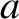; the time of perihelion passage,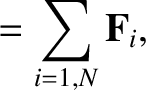; the eccentricity,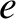; the inclination (to the ecliptic plane),; the argument of the perihelion,; and the longitude of the ascending node,. [The mean orbital angular velocity, in radians per year, is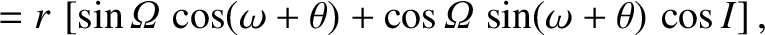, whereis measured in astronomical units. Here, an astronomical unit is the mean Earth-Sun distance, and corresponds to(Yoder 1995).]

In low-inclination orbits, the argument of the perihelion is usually replaced by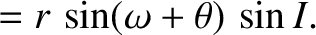(4.75)

which is termed the longitude of the perihelion. Likewise, the time of perihelion passage,, is often replaced by the mean longitude at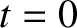--otherwise known as the mean longitude at epoch--where the mean longitude is defined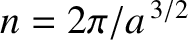(4.76)

Thus, if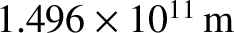denotes the mean longitude at epoch () then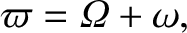(4.77)

where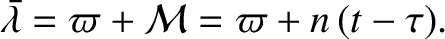. Another common alternative tois the mean anomaly at epoch,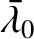(4.78)

The orbital elements of the major planets at the epoch J2000 (i.e., at 00:00 UT on January 1, 2000) are given in Table 4.1.

1pt

Table: Planetary data for J2000.- major radius;- mean longitude at epoch;- eccentricity;- inclination to ecliptic;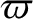- longitude of perihelion;- longitude of ascending node;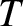- orbital period;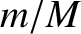- planetary mass / solar mass. Source: Standish and Williams (1992).
 Planet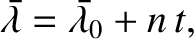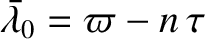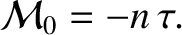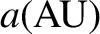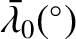T( yr)Mercury 0.3871 252.25 0.20564 7.006 77.46 48.34 0.241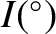Venus 0.7233 181.98 0.00676 3.398 131.77 76.67 0.615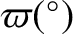Earth 1.0000 100.47 0.01673 0.000 102.93 - 1.000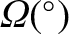Mars 1.5237 355.43 0.09337 1.852 336.08 49.71 1.881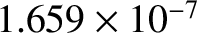Jupiter 5.2025 34.33 0.04854 1.299 14.27 100.29 11.87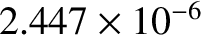Saturn 9.5415 50.08 0.05551 2.494 92.86 113.64 29.47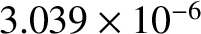Uranus 19.188 314.20 0.04686 0.773 172.43 73.96 84.05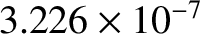Neptune 30.070 304.22 0.00895 1.770 46.68 131.79 164.9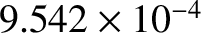The heliocentric (i.e., as seen from the Sun) position of a planet is most conveniently expressed in terms of its ecliptic longitude,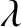, and ecliptic latitude,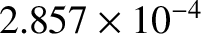. This type of longitude and latitude is referred to the ecliptic plane, with the Sun as the origin. Moreover, the vernal equinox is defined to be the zero of longitude. It follows that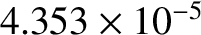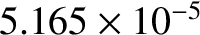(4.79) and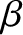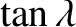(4.80)

where (,,) are the heliocentric Cartesian coordinates of the planet.Next: Planetary orbits Up: Keplerian orbits Previous: Elliptic orbits
Richard Fitzpatrick 2016-03-31